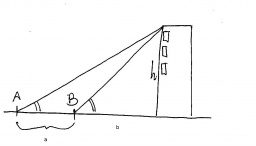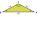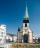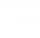# Angles of elevation

From points A and B on level ground, the angles of elevation of the top of a building are 25° and 37° respectively. If |AB| = 57m, calculate, to the nearest meter, the distances of the top of the building from A and B if they are both on the same side of the building.

Correct result:

h =  69.73 m
d1 =  165 m
d2 =  116 m

#### Solution:We would be very happy if you find an error in the example, spelling mistakes, or inaccuracies, and please send it to us. We thank you!Tips to related online calculators
Do you want to round the number?

#### You need to know the following knowledge to solve this word math problem:

We encourage you to watch this tutorial video on this math problem:

## Next similar math problems:

• Triangle in a squareIn a square ABCD with side a = 6 cm, point E is the center of side AB and point F is the center of side BC. Calculate the size of all angles of the triangle DEF and the lengths of its sides.
• TreeBetween points A and B is 50m. From A we see a tree at an angle 18°. From point B we see the tree in three times bigger angle. How tall is a tree?
• Ratio iso triangleThe ratio of the sides of an isosceles triangle is 7:6:7 Find the base angle to the nearest answer correct to 3 significant figure.
• Space diagonal anglesCalculate the angle between the body diagonal and the side edge c of the block with dimensions: a = 28cm, b = 45cm and c = 73cm. Then, find the angle between the body diagonal and the plane of the base ABCD.
• The rescue helicopterThe rescue helicopter is above the landing site at a height of 180m. The site of the rescue operation can be seen from here at a depth angle of 52° 40 '. How far will the helicopter land from the rescue site?
• Tetrahedral pyramidDetermine the surface of a regular tetrahedral pyramid when its volume is V = 120 and the angle of the sidewall with the base plane is α = 42° 30´.
• The aspect ratioThe aspect ratio of the rectangular triangle is 13: 12: 5. Calculate the internal angles of the triangle.
• Roof angleThe roof of the house has the shape of an isosceles triangle with arms 4 m long and the size of the base 6 m. How big an angle alpha does its roof make?
• Right triangleCalculate the missing side b and interior angles, perimeter and area of ​​a right triangle if a=10 cm and hypotenuse c = 16 cm.
• Trapezoid MOThe rectangular trapezoid ABCD with right angle at point B, |AC| = 12, |CD| = 8, diagonals are perpendicular to each other. Calculate the perimeter and area of ​​the trapezoid.
• Two forcesTwo forces with magnitudes of 25 and 30 pounds act on an object at angles of 10° and 100° respectively. Find the direction and magnitude of the resultant force. Round to two decimal places in all intermediate steps and in your final answer.
• Church towerArchdeacon church in Usti nad Labem has diverted tower by 186 cm. The tower is 65 m high. Calculate the angle by which the tower is tilted. Result write in degree's minutes.
• IS triangleCalculate interior angles of the isosceles triangle with base 40 cm and legs 22 cm long.
• Regular 5-gonCalculate area of the regular pentagon with side 7 cm.
• Coordinates of square verticesI have coordinates of square vertices A / -3; 1/and B/1; 4 /. Find coordinates of vertices C and D, C 'and D'. Thanks Peter.
• Sphere in coneA sphere of radius 3 cm desribe cone with minimum volume. Determine cone dimensions.
• Q-ExamIf tg α = 0.9, Calculating sin α, cos α, cotg α .